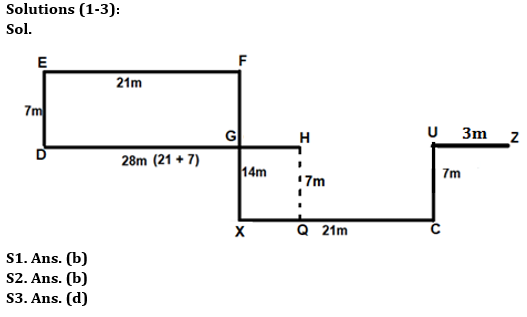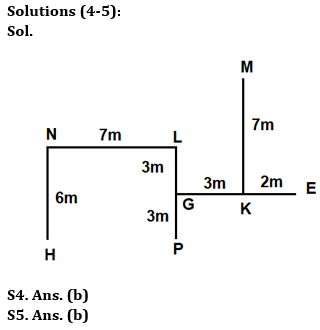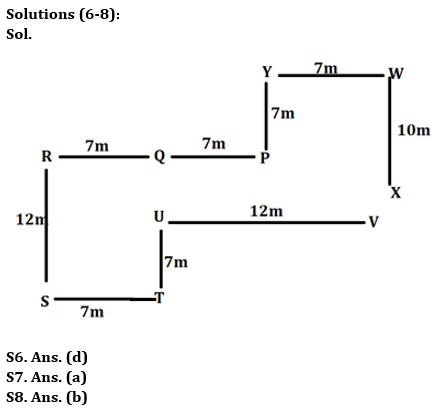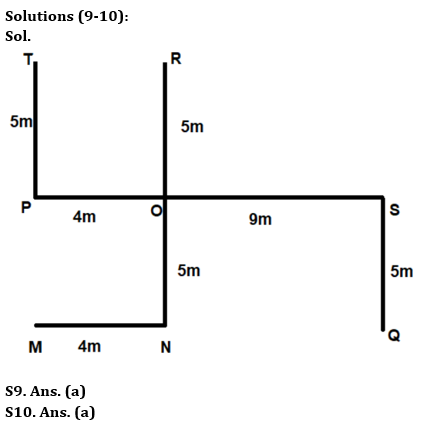Latest Banking jobs   »

# Reasoning Quizzes Quiz For IBPS RRB PO/Clerk Prelims 2023-24th May

Directions (1-3): Answer the questions based on the information given below.
Point U is 7m north of point C which is 21m east of point X. Point D is 7m south of point E which is 21m west of point of F. Point F is 14m north of point X. Point H is 28m east of point D. Point G is the midpoint between point F and point X. Point Z is in 3m east of point U.

Q1. If point Q is 7m south of point H then which of the following statement is true?
(a) Point Q is in west of point X
(b) Point Q is 14m away from point C
(c) Point Q is north-west of point F
(d) Point Q is south-west of point E
(e) None of the above

Q2. What is the direction of point Z with respect of X?
(a) North-west
(b) North-east
(c) South-west
(d) South-east
(e) None of the above

Q3. What is the direction of point C with respect of E?
(a) North-west
(b) North-east
(c) South-west
(d) South-east
(e) None of the above

Directions (4-5): Answer the questions based on the information given below.
Eight points (E, G, H, K, L, M, N, and P) are at different directions and distances with respect to one another. Point G is 3m west of Point K which is 7m south of Point M. Point E is 5m east of Point G which is 3m north of Point P. Point L is 3m north of Point G. Point L is 7m east of Point N which is 6m north of Point H.

Q4. The distance between point P and point H is same as the distance between point K and point _____.
(a) G
(b) M
(c) N
(d) L
(e) None of the above

Q5. What is the direction of point E with respect of point H?
(a) North-west
(b) North-east
(c) South-west
(d) South-east
(e) None of the above

Directions (6-8): Answer the questions based on the information given below.
A certain number of people sit in the stadium at some distance from each other. P is 7m east of Q. R is 7m west of Q. S is 12m south of R. Y is 7m north of P. T is 7m east of S. U is 7m north of T. U is 12m west of V. W is 7m east of Y. X is 10m south of W.

Q6. What is direction of S with respect to W?
(a) North-east
(b) North-west
(c) South-east
(d) South-west
(e) None of the above

Q7. What is the shortest distance between Q and U?
(a) 5m
(b) 6m
(c) 7m
(d) 8m
(e) None of the above

Q8. What is the direction of R with respect to X?
(a) North-east
(b) North-west
(c) South-east
(d) South-west
(e) None of the above

Directions (9-10): Study the following information carefully and answer the questions accordingly.
8 friends sit in a certain arrangement in a park. M sits 4m to the west of N. N sits 10m to the south of R. P sits 4m to the west of O. T sits 5m to the north of P. Q sits 5m to the south of S. O sits exactly in the middle of R and N. S sits 9m to the east of O.

Q9. What is the shortest distance and direction of Q with respect of M?
(a) 13m, east
(b) 14m, south
(c) 15m, west
(d) 13m, west
(e) None of these

Q10. What is the direction of T with respect to S?
(a) North-west
(b) South-east
(c) North-east
(d) South-west
(e) None of these

Solutions## FAQs

### What is the selection process of IBPS RRP PO?

There are 4 sections in the IRDAI assistant manager online preliminary exam. They are Reasoning, English Language, General awareness, Quantitative aptitude.

#### Congratulations!Union Budget 2023-24: Free PDF